Next: Exercises Up: Addition of Angular Momentum Previous: Clebsch-Gordon Coefficients

# Calculation of Clebsch-Gordon Coefficients

Let us add the angular momenta of two spin one-half systems. For example, two electrons at rest. So,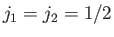. We know, from general principles, that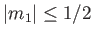and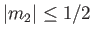. We also know, from Equation (6.26), that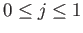, where the allowed values of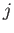differ by integer amounts. It follows that either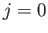or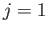. Thus, two spin one-half systems can be combined to form either a spin-zero system or a spin-one system. It is helpful to arrange all of the possibly non-zero Clebsch-Gordon coefficients in a table: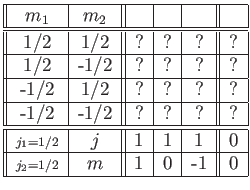The box in this table corresponding to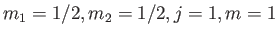gives the Clebsch-Gordon coefficient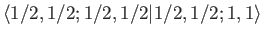, or the inverse Clebsch-Gordon coefficient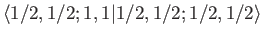. All the boxes contain question marks because, at this stage, we do not know the values of any Clebsch-Gordon coefficients.

A Clebsch-Gordon coefficient is automatically zero unless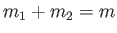. In other words, the-components of angular momentum have to add algebraically. Many of the boxes in the previous table correspond to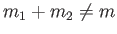. We immediately conclude that these boxes must contain zeroes. Thus,The normalization condition (6.28) implies that the sum of the squares of all the rows and columns of the previous table must be unity. There are two rows and two columns that contain only a single non-zero entry. We conclude that these entries must be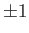, but we have no way of determining the signs at present. Thus,Let us evaluate the recursion relation (6.32) for, with,,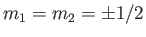, taking the upper/lower sign. We find that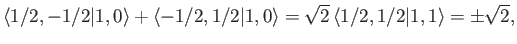(6.32)

and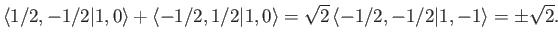(6.33)

Here, the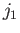and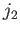labels have been suppressed for ease of notation. We also know that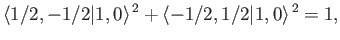(6.34)

from the normalization condition. The only real solutions to the previous set of equations are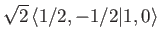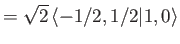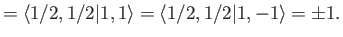(6.35)

The choice of sign is arbitrary--the conventional choice is a positive sign. Thus, our table now readsWe could fill in the remaining unknown entries of our table by using the recursion relation again. However, an easier method is to observe that the rows and columns of the table must all be mutually orthogonal. That is, the dot product of a row with any other row must be zero. Likewise, for the dot product of a column with any other column. This follows because the entries in the table give the expansion coefficients of one of our alternative sets of eigenkets in terms of the other set, and each set of eigenkets contains mutually orthogonal vectors with unit norms. The normalization condition tells us that the dot product of a row or column with itself must be unity. The only way that the dot product of the fourth column with the second column can be zero is if the unknown entries are equal and opposite. The requirement that the dot product of the fourth column with itself is unity tells us that the magnitudes of the unknown entries have to be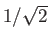. The unknown entries are undetermined to an arbitrary sign multiplied into them both. Thus, the final form of our table (with the conventional choice of arbitrary signs) isThe table can be read in one of two ways. The columns give the expansions of the eigenstates of overall angular momentum in terms of the eigenstates of the individual angular momenta of the two component systems. Thus, the second column tells us that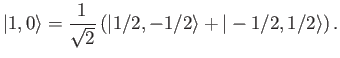(6.36)

The ket on the left-hand side is a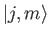ket, whereas those on the right-hand side are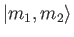kets. The rows give the expansions of the eigenstates of individual angular momentum in terms of those of overall angular momentum. Thus, the second row tells us that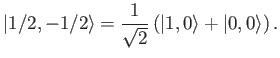(6.37)

Here, the ket on the left-hand side is aket, whereas those on the right-hand side arekets.

Note that our table is really a combination of two sub-tables, one involvingstates, and one involvingstates. The Clebsch-Gordon coefficients corresponding to two different choices ofare completely independent. That is, there is no recursion relation linking Clebsch-Gordon coefficients corresponding to different values of. Thus, for every choice of,, andwe can construct a table of Clebsch-Gordon coefficients corresponding to the different allowed values of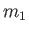,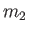, and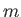(subject to the constraint that). A complete knowledge of angular momentum addition is equivalent to knowing all possible tables of Clebsch-Gordon coefficients. These tables are listed (for moderate values of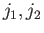and) in many standard reference books and articles .Next: Exercises Up: Addition of Angular Momentum Previous: Clebsch-Gordon Coefficients
Richard Fitzpatrick 2016-01-22